# 大學物理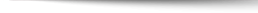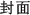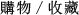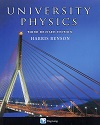## University Physics Third Revised Edition

+作者：

### Benson

+年份：
2019 年Third Revised 版
+ISBN：
9789867696250
+書號：
PH0163PC
+規格：

+頁數：
1008
+出版商：
Benson
+參考網址：

\$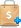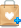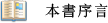The main change in this Revised Edition of University Physics is the addition of about 550 new exercises and a few new problems. The "Additional Exercises" are placed at the end of the original exercises, which have been left unchanged. In response to the suggestions of users of the first edition, the new exercises are not keyed to the sections in each chapter. There are now about 2440 exercises and 560 problems, for a total of about 3000. I have taken the opportunity to include some minor clarifications and updating of the text. Despite these changes, the length of the book has increased only marginally to 942 pages. The text is now available in cloth and paperback.Accuracy
My primary objective has been to present concepts and principles clearly and correctly. I hope that the physics is free of even subtle misconceptions. In some optional sections I try to deal correctly with topics, such as the work-energy theorem for systems, that are inadequately treated in other texts. Attention is given to details such as the subtle question of signs in the application of Coulomb's law, in Faraday's law, or in Kirchhoff's loop rule for ac circuits. The concepts of emf and potential difference are clearly distinguished. The acceleration due to gravity and the gravitational field strength are given different symbols (although I do not dwell on this distinction).
Writing Style
I have tried to write in a simple, clear, and concise manner. This approach applies as much to the language as to the presentation of the mathematics and the notation used. The examples emphasize the important, or conceptually difficult, steps. Although the coverage is fairly complete, this textbook is significantly shorter than many that have appeared in recent years.
Pedagogy
I focus on central issues and highlight as few equations as possible (in the second color). Special cases, such as the "range formula" in projectile motion, are often discussed in an example and do not appear in the chapter summary. Also, I prefer not to present multiple versions of the same equation. For example, the intensity variation in the double-slit interference pattern appears only in terms of the phase difference (cf>), and not in terms of the angular position (O) or the vertical coordinate on the screen (y).
Questions, Exercises, and Problems
There is a large selection of questions, exercises, and problems. The questions are limited to those that students should find useful in improving their understanding. I avoid questions with no clear-cut answers. The exercises are keyed to the sections, whereas the problems are not. As an aid to students and instructors, both the exercises and the problems are graded according to two levels of difficulty: I and II. The answers to the odd-numbered exercises and problems are given in the text. A solutions manual containing brief solutions for all the exercises and problems is available only to instructors, not to students.
Content and Structure
The text includes almost all the traditional topics in classical physics. The last six chapters cover selected topics in modern physics. Basic material appears in a single-column format, whereas optional sections are in a two-column format. The overall structure is conventional. The discussions of the dot and cross products fit neatly into Chapter 2 but are easily postponed until they are needed. Chapters 15 to 17 on oscillations and waves may be combined with optics for a unified treatment of waves. The dynamics and energy aspects of satellite motion are discussed in Chapters 6 and 8, respectively. These topics may be delayed until Chapter 13 for a unified treatment of gravitation, but, as it stands, the whole of Chapter 13 may be omitted.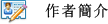Harris Benson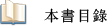Chapter 1 INTRODUCTION
Chapter 2 VECTORS
Chapter 3 ONE-DIMENSIONAL KINEMATICS
Chapter 4 INERTIA AND TWO DIMENSIONAL MOTION
Chapter 5 PARTICLE DYNAMICS I
Chapter 6 PARTICLE DYNAMICS II
Chapter 7 WORK AND ENERGY
Chapter 8 CONSERVATION OF MECHANICAL ENERGY
Chapter 9 LINEAR MOMENTUM
Chapter 10 SYSTEMS OF PARTICLES
Chapter 11 ROTATION OF A RIGID BODY ABOUT A FIXED AXIS
Chapter 12 ANGULAR MOMENTUM AND STATICS
Chapter 13 GRAVITATION
Chapter 14 SOLIDS AND FLUIDS
Chapter 15 OSCILLATIONS
Chapter 16 MECHANICAL WAVES
Chapter 17 SOUND
Chapter 18 TEMPERATURE, THERMAL EXPANSION, AND THE IDEAL GAS LAW
Chapter 19 FIRST LAW OF THERMODYNAMICS
Chapter 20 KINETIC THEORY
Chapter 21 ENTROPY AND THE SECOND LAW OF THERMODYNAMICS
Chapter 22 ELECTROSTATICS
Chapter 23 THE ELECTRIC FIELD
Chapter 24 GAUSS'S LAW
Chapter 25 ELECTRIC POTENTIAL
Chapter 26 CAPACITORS AND DIELECTRICS
Chapter 27 CURRENT AND RESISTANCE
Chapter 28 DIRECT CURRENT CIRCUITS
Chapter 29 THE MAGNETIC FIELD
Chapter 30 SOURCES OF THE MAGNETIC FIELD
Chapter 31 ELECTROMAGNETIC INDUCTION
Chapter 32 INDUCTANCE AND MAGNETIC MATERIALS
Chapter 33 ALTERNATING CURRENT CIRCUITS
Chapter 34 MAXWELL'S EQUATIONS; ELECTROMAGNETIC WAVES
Chapter 35 LIGHT: REFLECTION AND REFRACTION
Chapter 36 LENSES AND OPTICAL INSTRUMENTS
Chapter 37 WAVE OPTICS (I)
Chapter 38 WAVE OPTICS (II)
Chapter 39 SPECIAL RELATIVITY
Chapter 40 EARLY QUANTUM THEORY
Chapter 41 WAVE MECHANICS
Chapter 42 ATOMS AND SOLIDS
Chapter 43 NUCLEAR PHYSICS
Chapter 44 ELEMENTARY PARTICLES
APPENDICES
A SI Units
B Mathematics Review
C Calculus Review
D The Periodic Table
E Table of Isotopes
ANSWERS TO ODD-NUMBERED EXERCISES AND PROBLEMS